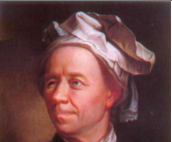## 26 August 2010

### Project Euler : Discover all the fractions with an unorthodox cancelling method.Problem 33

The fraction49/98is a curious fraction, as an inexperienced mathematician in attempting to simplify it may incorrectly believe that49/98=4/8, which is correct, is obtained by cancelling the 9s.
We shall consider fractions like,30/50=3/5, to be trivial examples.
There are exactly four non-trivial examples of this type of fraction, less than one in value, and containing two digits in the numerator and denominator.
If the product of these four fractions is given in its lowest common terms, find the value of the denominator.

Solution (in Ruby)

This problem unlike the other problems does not ask us to go to millions and trillions and the scope is such that this can be solved in the least running time. All this problem demands careful inspection of the problem statement itself. Its clear we need to iterate through numbers from 1 to 99. For the outer loop (numerator), its enough we run from 12 since its the first double digit number which does not end with a 0 or their digits being equal. We run upto 97 since the numerator cannot be greater than equal to denominator since the fraction should be less than 1
Same is the case for the inner loop. We should always continue to the next iteration if the last number of either numerator or denominator is 0, or if both digits or equal or if the numerator is greater than or equal to denominator. Rest of the solution is just a language specific implementation of finding whether the two different fractions are indeed equal.

Hover here to see the solution

Cheers!!